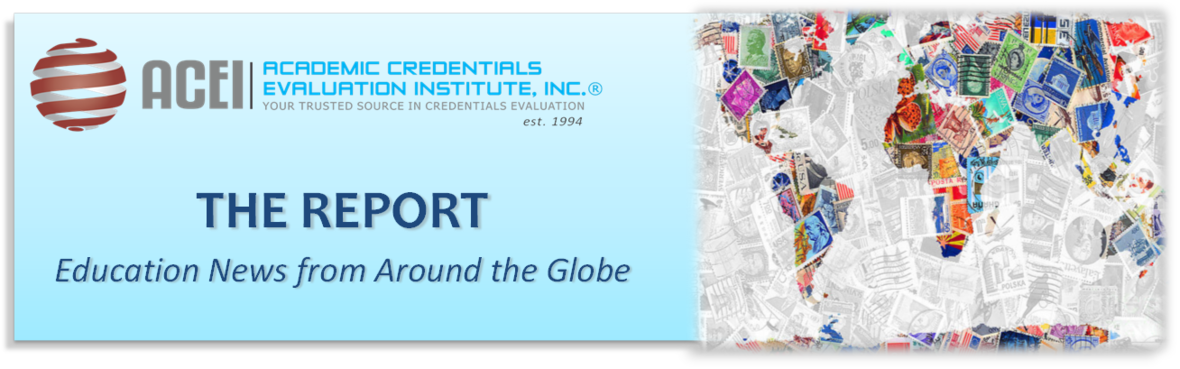# May 2018 - Beverly Hills, CA

ACADEMIC CREDENTIALS EVALUATION INSTITUTE, INC. (ACEI)
Is incorporated in the state of California (1994), and is dedicated to the advancement of international academic exchange and understanding through the dissemination of information on international academic credentials.

 table div table+table+table div table{width:100%;padding:0}table div table+table+table div table img{width:96.23%;padding:0;float:none}table div table+table+table div table td{width:100%;padding:0 1.88% 18px}/* styles */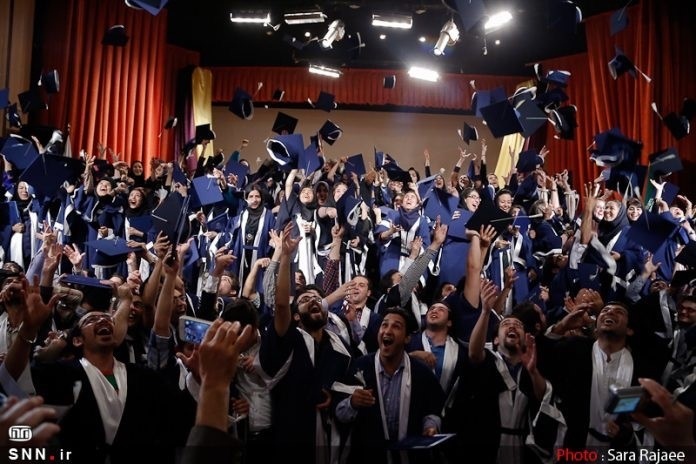## IRAN

Although the US move to withdraw from the Iran nuclear deal may have no impact on the educational status of Iranian students studying abroad, universities in Iran are completely ready to admit them if they decide to return, said Iran’s Deputy Minister for Science, Research and Technology. Iran Front Page reports:

 table div table+table+table+table+table+table div table{width:100%;padding:0}table div table+table+table+table+table+table div table img{width:96.23%;padding:0;float:none}table div table+table+table+table+table+table div table td{width:100%;padding:0 1.88% 18px}/* styles */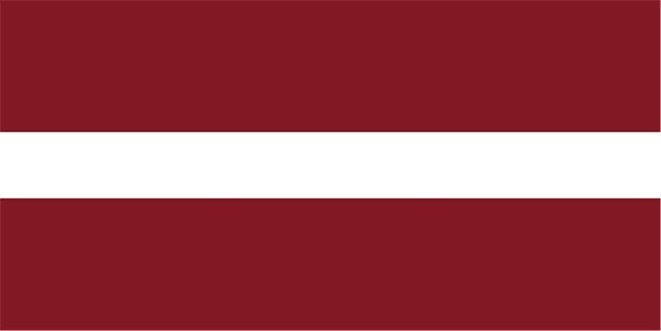# MOE proposes a ban on teaching in Russian at private universities

The Education and Science Ministry is proposing applying to private universities and colleges the same restrictions that apply to state higher education institutions, where students have to be taught in Latvian or any of the official languages of the European Union, which means that private colleges and universities would not be permitted to teach their students in Russian. Baltic Times reports:

 table div table+table+table+table+table+table+table+table div table{width:100%;padding:0}table div table+table+table+table+table+table+table+table div table img{width:96.23%;padding:0;float:none}table div table+table+table+table+table+table+table+table div table td{width:100%;padding:0 1.88% 18px}/* styles */# International Education is Key to Diplomacy

International students enhance "the quality of education for the college’s domestic students, by bringing them into direct contact with students from other countries.” The Pie News reports:

 table div table+table+table+table+table+table+table+table+table+table+table div table{width:100%;padding:0}table div table+table+table+table+table+table+table+table+table+table+table div table img{width:96.23%;padding:0;float:none}table div table+table+table+table+table+table+table+table+table+table+table div table td{width:100%;padding:0 1.88% 18px}/* styles */# Universities in court for offering ‘too many courses in English’

A lobby group campaigning for better education in the Netherlands is taking Maastricht and Twente universities to court for offering too many degree courses in English. Dutch News reports:

 table div table+table+table+table+table+table+table+table+table+table+table+table+table+table div table{width:100%;padding:0}table div table+table+table+table+table+table+table+table+table+table+table+table+table+table div table img{width:96.23%;padding:0;float:none}table div table+table+table+table+table+table+table+table+table+table+table+table+table+table div table td{width:100%;padding:0 1.88% 18px}/* styles */# Nigeria Set to Open yet Another Military University

A new military university focused on nuclear technology is to be located in the town of Biu in Borno State, a uranium-rich area and the epicentre of the Boko Haram insurgency which has ravaged the country for the last eight years. While the new institution will be open to civilians, not everyone agrees that Nigeria needs another military university. University World News Reports:

 table div table+table+table+table+table+table+table+table+table+table+table+table+table+table+table+table+table div table{width:100%;padding:0}table div table+table+table+table+table+table+table+table+table+table+table+table+table+table+table+table+table div table img{width:96.23%;padding:0;float:none}table div table+table+table+table+table+table+table+table+table+table+table+table+table+table+table+table+table div table td{width:100%;padding:0 1.88% 18px}/* styles */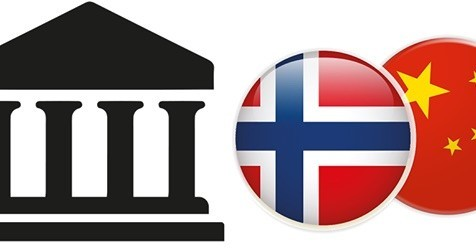# Norway and China: Education Partners

Norway is forging ahead with strengthening its education partnership with China. NOKUT reports:

 table div table+table+table+table+table+table+table+table+table+table+table+table+table+table+table+table+table+table+table div table{width:100%;padding:0}table div table+table+table+table+table+table+table+table+table+table+table+table+table+table+table+table+table+table+table div table img{width:96.23%;padding:0;float:none}table div table+table+table+table+table+table+table+table+table+table+table+table+table+table+table+table+table+table+table div table td{width:100%;padding:0 1.88% 18px}/* styles */# KP Government announces construction of 10 New Universities

Khyber Pakhtunkhwa Higher Education Department has successfully constructed 10 additional universities, including two women universities during its tenure. Pakistan Observer reports:

 table div table+table+table+table+table+table+table+table+table+table+table+table+table+table+table+table+table+table+table+table+table div table{width:100%;padding:0}table div table+table+table+table+table+table+table+table+table+table+table+table+table+table+table+table+table+table+table+table+table div table img{width:96.23%;padding:0;float:none}table div table+table+table+table+table+table+table+table+table+table+table+table+table+table+table+table+table+table+table+table+table div table td{width:100%;padding:0 1.88% 18px}/* styles */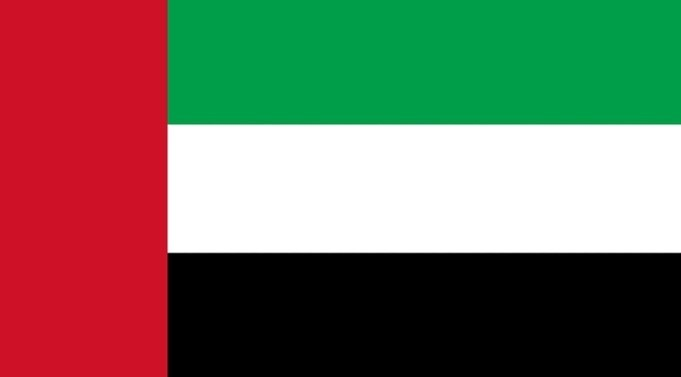# UAE extends student visa to attract more international students

The new visa policy for students enables a 5-year residency visa for all students and a 10-year-visa for academically exceptional one. Khaleej Times reports:

 table div table+table+table+table+table+table+table+table+table+table+table+table+table+table+table+table+table+table+table+table+table+table+table div table{width:100%;padding:0}table div table+table+table+table+table+table+table+table+table+table+table+table+table+table+table+table+table+table+table+table+table+table+table div table img{width:96.23%;padding:0;float:none}table div table+table+table+table+table+table+table+table+table+table+table+table+table+table+table+table+table+table+table+table+table+table+table div table td{width:100%;padding:0 1.88% 18px}/* styles */# Where have all the international students gone?

We hear a great deal about the drop in international student numbers at U.S. institutions. In her recent blog for #ACEI-Global.blog, ACEI President & CEO, Jasmin Saidi-Kuehnert writes about this issue and also includes examples of some of the positive actions U.S. HEIs are taking to remain a study abroad destination for international students.

 table div table+table+table+table+table+table+table+table+table+table+table+table+table+table+table+table+table+table+table+table+table+table+table+table+table div table{width:100%;padding:0}table div table+table+table+table+table+table+table+table+table+table+table+table+table+table+table+table+table+table+table+table+table+table+table+table+table div table img{width:96.23%;padding:0;float:none}table div table+table+table+table+table+table+table+table+table+table+table+table+table+table+table+table+table+table+table+table+table+table+table+table+table div table td{width:100%;padding:0 1.88% 18px}/* styles */# Bilateral Seminar: Transatlantic Friendship & Mobility Initiative

ACEI President & CEO, Jasmin Saidi-Kuehnert, and President of the AICE (Association of International Credential Evaluators), reports on the May 14-15 Bilateral Seminar on Franco-USA education exchange held at the Embassy of France in Washington, DC. For a summary of the report, click here. For a full report, click here.

 table div table+table+table+table+table+table+table+table+table+table+table+table+table+table+table+table+table+table+table+table+table+table+table+table+table+table+table div table{width:100%;padding:0}table div table+table+table+table+table+table+table+table+table+table+table+table+table+table+table+table+table+table+table+table+table+table+table+table+table+table+table div table img{width:96.23%;padding:0;float:none}table div table+table+table+table+table+table+table+table+table+table+table+table+table+table+table+table+table+table+table+table+table+table+table+table+table+table+table div table td{width:100%;padding:0 1.88% 18px}/* styles */## ACEI NEWS

 table.module-28{width:84.72%;padding:0}table div table+table+table+table+table+table+table+table+table+table+table+table+table+table+table+table+table+table+table+table+table+table+table+table+table+table+table+table+table div table{width:84.72%;float:none;margin-left:auto;margin-right:auto;padding:0}table div table+table+table+table+table+table+table+table+table+table+table+table+table+table+table+table+table+table+table+table+table+table+table+table+table+table+table+table+table div table a{border:0 none;text-decoration:none}table div table+table+table+table+table+table+table+table+table+table+table+table+table+table+table+table+table+table+table+table+table+table+table+table+table+table+table+table+table div table img{width:100%!important;border:0 none;text-decoration:none}table div table+table+table+table+table+table+table+table+table+table+table+table+table+table+table+table+table+table+table+table+table+table+table+table+table+table+table+table+table div table td{width:100%;padding:0}/* styles */

# Digital Delivery of Evaluation Reports: SecurePathway©:

ACEI is a proud signatory of the Groningen Declaration Network which “seeks common ground in best serving the academic and professional mobility needs of citizens worldwide by bringing together key stakeholders in the Digital Student Data Ecosystem.” For the past two years, ACEI has committed itself to this shared mission through the introduction of SecurePathway©, an ACEI proprietary digital platform for the secure delivery of official evaluation reports and supporting educational credentials. For information on ACEI’s SecurePathway©.

Signing up with ACEI’s SecurePathway©, is free and does not cost the institutions any fees. To receive ACEI expert reports and certified academic credentials via SecurePathway©, complete this form.

*SecurePathwaysRegistrationForm*/* styles */ ACEI’s Advisory Services provide institutions and organizations looking at expanding their international student recruitment with customized solutions to help overcome enrollment challenges, uncover opportunities, and devise an innovative and holistic approach to international enrollment. For more information on ACEI’s Advisory Services and how we can assist you, please contact Yolinisse Moreno, Director of Programs & Communications at ymoreno@acei-global.org.
 table div table+table+table+table+table+table+table+table+table+table+table+table+table+table+table+table+table+table+table+table+table+table+table+table+table+table+table+table+table+table+table+table+table div table{width:100%;padding:0}table div table+table+table+table+table+table+table+table+table+table+table+table+table+table+table+table+table+table+table+table+table+table+table+table+table+table+table+table+table+table+table+table+table div table img{width:96.23%;padding:0;float:none}table div table+table+table+table+table+table+table+table+table+table+table+table+table+table+table+table+table+table+table+table+table+table+table+table+table+table+table+table+table+table+table+table+table div table td{width:100%;padding:0 1.88% 18px}/* styles */## Upcoming Conferences & Presentations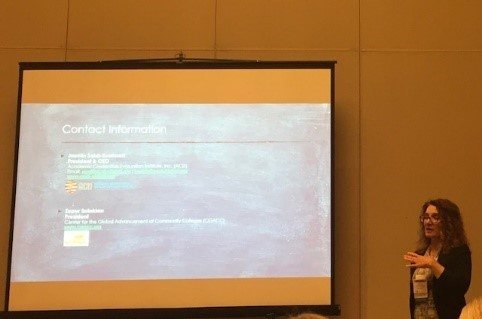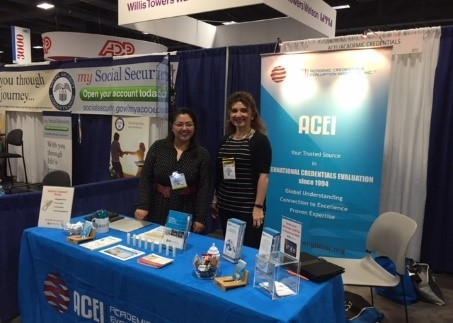## ACEI will be attending the following conference in 2018 and hope you will have a chance to attend the session presented by our representatives:

Open Meeting: Outsourcing Credential Evaluations
ACEI President & CEO, Jasmin Saidi-Kuehnert together with Aleks Morawski, Foreign Credits, will be facilitating the “Open Meeting: Credentials Town Hall: In-House Versus Outsourcing Credentialing” at the NAFSA Conference.
Date: Wednesday, May 30
Time: 8:30 AM - 9:30 AM
Location: PCC, Room 121A

AICE Coffee Hour Meetup
Interested in learning about AICE and its Endorsed Members? Considering to join as an Affiliate? Come meet with AICE Endorsed Member representatives, Jasmin Saidi-Kuehnert (ACEI) and Aleks Morawski (Foreign Credits) and take part in drawing. You may just be the winner of the \$300 travel grant to AICE’s 2019 Symposium in Los Angeles, CA.
Date: Thursday, May 31
Time: 3:00 PM – 4:00 PM
Location: Panera Bakery, 1201 Arch Street Panera Bakery, Philadelphia, PA

 table div table+table+table+table+table+table+table+table+table+table+table+table+table+table+table+table+table+table+table+table+table+table+table+table+table+table+table+table+table+table+table+table+table+table+table+table+table div table{width:100%;padding:0}table div table+table+table+table+table+table+table+table+table+table+table+table+table+table+table+table+table+table+table+table+table+table+table+table+table+table+table+table+table+table+table+table+table+table+table+table+table div table img{width:96.23%;padding:0;float:none}table div table+table+table+table+table+table+table+table+table+table+table+table+table+table+table+table+table+table+table+table+table+table+table+table+table+table+table+table+table+table+table+table+table+table+table+table+table div table td{width:100%;padding:0 1.88% 18px}/* styles *//* styles */ ACEI offers a comprehensive range of professional services that include: international credential evaluations; translations; consulting; and training. For more information on ACEI, please visit www.acei-global.org or contact: Tel: 1-310-275-3530; Email: acei@acei-global.org. ACEI was founded and incorporated in 1994 in the State of California and is a Charter and Endorsed Member of the Association of International Credential Evaluators (AICE).
 Like   Tweet   Pin   +1   in